MORE IN Analog and Digital Electronics
VTU Computer Science (Semester 3)
Analog and Digital Electronics
June 2012
Total marks: --
Total time: --
INSTRUCTIONS
(1) Assume appropriate data and state your reasons
(2) Marks are given to the right of every question
(3) Draw neat diagrams wherever necessary

1 (a) Explain the effects of collector resistor, base current and supply voltage on the operating point of a fixed bias circuit. Which is the ideal position for an operating point on the BJT fixed bias transistor circuit? Explain the above with neat daigrams.
10 M
1 (b) What is the operating point for the following voltage divider bias circuit?
Assume β=150
VBE=0.7 V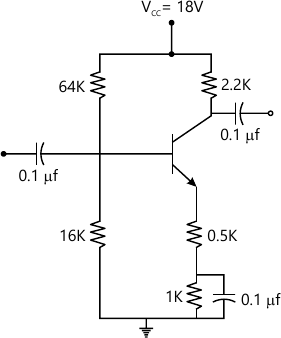10 M

2 (a) Explain the working of N-channel E-Mosfet with neat diagram. Explain with a diagram output characteristics of the same.
10 M
2 (b) Find the values of voltages VD and VC for the circuit shown, Fig. Q2(b). Assume β=100, VBE=0.7 V, saturation drain current of JFET is -10 mA and pinch off voltage is- 5V.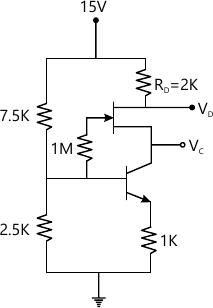10 M

3 (a) Explain photodiode, photosensor, photo conductor and phototransistors with necessary diagrams.
10 M
3 (b) Find the value of RL for the circuit shown, Fig. Q3(b) such that the circuit gives a logic high when the light incident on it is above 200 lux and the photo condutor has a resistance of 14 KΩ at a light level of 100 lux, α=0.5, power supply voltage is VCC = 10V and reference voltage of zener diode is 3.5V.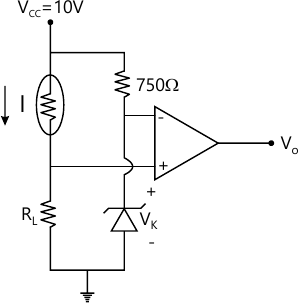10 M

4 (a) Graphically how h-parameter of a BJT are determined? Explain with neat diagram. Also derive expression for input impedance and voltage gain for a BJT amplifier.
10 M
4 (b) Give the hybrid equivalent model for the circuit shown, in Fig.Q4(b). Find input impedance, voltage gain, current gain and output impedance. The h-parameters are the hie-1.5 k, hje=100, hre=1×10-4, hoe=25 μ A/V.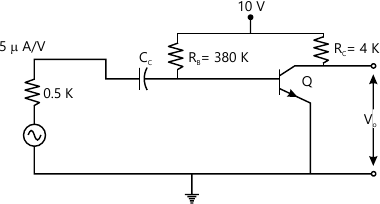10 M

5 (a) Discuss large signal amplifier characteristics. Discuss harmonic distrotion. Derive A0, A1, A2, A3, A4 the amplitude of D,C, first, second, third, forth amplitude of harmonic components.
10 M
5 (b) Derive expression for gain, input resistance and output resistance of voltage shunt feedback amplifier with the help of neat diagram. For the opamp based inverting amplifier circuit shown in Fig.Q5(b) find input impedance given that transimpedance, input impedance and output impedance of opamp are 100 MΩ, 10 M&OMega; and 100 Ω respectively.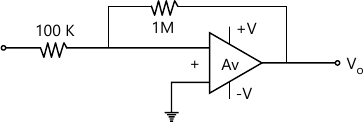10 M

6 (a) Mention the conditions necessary for oscillations in a feddback amplifier circuit. Determine the frequency at which the following circuit, shown in FIG.Q6(a) would oscillate if the loop gain criterion was met.
Also determine the maximum value of R1 for sustained oscillation.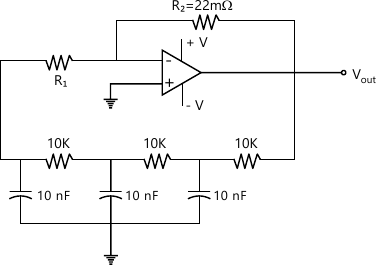10 M
6 (b) With a neat diagram and waveforms explain the working of a bistable multivibrator. (BJT based).
10 M

7 (a) Explain regulated power supply parameters: Load regulation, line regulation, output impedance, Ripple rejection factor. Determine the output ripple of a regulated power supply which provides a ripple rejection of-20dB and a ripple voltage in the unregulated input were 2V.
10 M
7 (b) Explain buck regulator boost regulator and inverting regulator with neat diagram.
10 M

8 (a) Explain with neat diagram:
(i) Peak detector circuit
(ii) Absolute value circuit and their working.
10 M
8 (b) Explain with neat diagram:
(i) Current to voltage converter
(ii) Voltage to current converter and their working.
10 M

More question papers from Analog and Digital Electronics